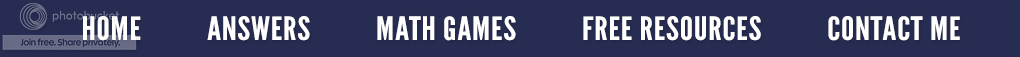### The Left Angle Mystery - Does Such an Angle Exist?

Geometry is probably my favorite part of math to teach because it is so visual; plus the subject lends itself to doing many hands-on activities, even with my college students.  When our unit on points, lines and angles is finished, it is time for the unit test.  Almost every year I ask the following question:  What is a left angle?   Much to my chagrin, here are some of the responses I have received over the years.1)   A left angle is the opposite of a right angle.

2)  On a clock, 3:00 o'clock is a right angle, but 9:00 o'clock is a left angle.

3)  A left angle is when the base ray is pointing left instead of right.

4)      A left angle is 1/2 of a straight angle, like when it is cut into two pieces, only it is the part on the left, not the part on the right.
5)      A left angle is 1/4 of a circle, but just certain parts. Here is what I mean.

Now you know why math teachers, at times, want to pull their hair out!  Just to set the record straight, in case any of my students are reading this, there is no such thing as a left angle!  No matter which way the base ray is pointing, any angle that contains 90is called a right angle.Only \$3.25
If you would like some different hands-on ways to teach angles, you might look at the resource entitled, Angles: Hands-on Activities.